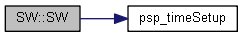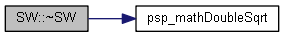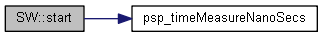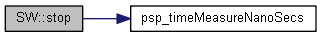SW Class Reference

`#include "sw.h"`

## Public Member Functions

SW (std::string description)
void start (void)
void stop (void)
~SW ()
SW (std::string description)
void start (void)
void stop (void)
~SW ()

## SW() [1/2]

 SW::SW ( std::string description )
inline
50 : n (0), sum (0), sum2 (0), min ((uint64_t) -1), max (0), description (description)
51 {
53 }

References psp_timeSetup().

Here is the call graph for this function:## ~SW() [1/2]

 SW::~SW ( )
inline
93 {
94using namespace std;
95double units = 1000000000;
96const double average = n ? (double) sum / units / n : 0.0;
97const double sigma = n > 1 ? ((double) sum2 / units / units - (double) sum / units / n * ((double) sum / units)) / (n - 1) : 0.0;
98const double sigma_corrected = sigma > 0.0 ? sigma : 0.0;
99const double standardDeviation = psp_mathDoubleSqrt (sigma_corrected);
100const double minimum = n ? (double) min / units : 0.0;
101const double maximum = (double) max / units;
102const double total = (double) sum / units;
103
104 cout << fixed << setprecision(9);
105 cout << (double) average << " +/- " << standardDeviation << " [" << minimum << ", " << maximum << "], n: " << setw(5) << n
106 << ", total: " << total << ", " << description << endl;
107 }

References psp_mathDoubleSqrt().

Here is the call graph for this function:## SW() [2/2]

 SW::SW ( std::string description )
inline
50 : n (0), sum (0), sum2 (0), m_min ((uint64_t) -1), m_max (0), description (description)
51 {
53 }

References psp_timeSetup().

Here is the call graph for this function:## ~SW() [2/2]

 SW::~SW ( )
inline
93 {
94using namespace std;
95double units = 1000000000;
96const double average = n ? (double) sum / units / n : 0.0;
97const double standardDeviation =
98 n > 2 ? psp_mathDoubleSqrt (((double) sum2 / units / units - (double) sum / units * sum / units / n) / (n - 1)) : 0.0;
99const double m_minimum = n ? (double) m_min / units : 0.0;
100const double m_maximum = (double) m_max / units;
101const double total = (double) sum / units;
102
103if (n > 0)
104 {
105 cout << fixed << setprecision(9);
106 cout << (double) average << " +/- " << standardDeviation << " [" << m_minimum << ", " << m_maximum << "], n: " << setw(4) << n
107 << ", total: " << total << ", " << description << endl;
108 }
109 }

References psp_mathDoubleSqrt().

Here is the call graph for this function:## start() [1/2]

 void SW::start ( void )
inline
Examples
cpp55Example_main.cpp.
61 {
62 stamp = psp_timeMeasureNanoSecs ();
63 }

References psp_timeMeasureNanoSecs().

Here is the call graph for this function:## start() [2/2]

 void SW::start ( void )
inline
61 {
62 stamp = psp_timeMeasureNanoSecs ();
63 }

References psp_timeMeasureNanoSecs().

Here is the call graph for this function:## stop() [1/2]

 void SW::stop ( void )
inline
Examples
cpp55Example_main.cpp.
73 {
74const uint64_t measurement = psp_timeMeasureNanoSecs () - stamp;
75 n++;
76if (measurement > max)
77 {
78 max = measurement;
79 }
80if (measurement < min)
81 {
82 min = measurement;
83 }
84 sum += measurement;
85 sum2 += measurement * measurement;
86 }

References psp_timeMeasureNanoSecs().

Here is the call graph for this function:## stop() [2/2]

 void SW::stop ( void )
inline
73 {
74const uint64_t measurement = psp_timeMeasureNanoSecs () - stamp;
75 n++;
76if (measurement > m_max)
77 {
78 m_max = measurement;
79 }
80if (measurement < m_min)
81 {
82 m_min = measurement;
83 }
84 sum += measurement;
85 sum2 += measurement * measurement;
86 }

References psp_timeMeasureNanoSecs().

Here is the call graph for this function:The documentation for this class was generated from the following file:
RDM_EXPORT double psp_mathDoubleSqrt(double dv)
RDM_EXPORT RDM_RETCODE psp_timeSetup(void)
RDM_EXPORT uint64_t psp_timeMeasureNanoSecs(void)
RDM_EXPORT RDM_RETCODE psp_timeSetup(void)
RDM_EXPORT double psp_mathDoubleSqrt(double dv)
RDM_EXPORT uint64_t psp_timeMeasureNanoSecs(void)